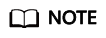Help Center > > Developer Guide> SQL Reference> Expressions> Array Expressions

# Array Expressions

Updated at:Mar 13, 2020 GMT+08:00

#### IN

expression IN (value [, ...])

The parentheses on the right contain an expression list. The expression result on the left is compared with the content in the expression list. If the content in the list meets the expression result on the left, the result of IN is true. If no result meets the requirements, the result of IN is false.

Example:

 ```1 2 3 4 5``` ```SELECT 8000+500 IN (10000, 9000) AS RESULT; result ---------- f (1 row) ```If the expression result is null or the expression list does not meet the expression conditions and at least one empty value is returned for the expression list on the right, the result of IN is null rather than false. This method is consistent with the Boolean rules used when SQL statements return empty values.

#### NOT IN

expression NOT IN (value [, ...])

The parentheses on the right contain an expression list. The expression result on the left is compared with the content in the expression list. If the content in the list does not meet the expression result on the left, the result of NOT IN is true. If any content meets the expression result, the result of NOT IN is false.

Example:

 ```1 2 3 4 5``` ```SELECT 8000+500 NOT IN (10000, 9000) AS RESULT; result ---------- t (1 row) ```If the query statement result is null or the expression list does not meet the expression conditions and at least one empty value is returned for the expression list on the right, the result of NOT IN is null rather than false. This method is consistent with the Boolean rules used when SQL statements return empty values.

In all situations, X NOT IN Y equals to NOT(X IN Y).

#### ANY/SOME (array)

expression operator ANY (array expression)

expression operator SOME (array expression)

 ```1 2 3 4 5``` ```SELECT 8000+500 < SOME (array[10000,9000]) AS RESULT; result ---------- t (1 row) ```
 ```1 2 3 4 5``` ```SELECT 8000+500 < ANY (array[10000,9000]) AS RESULT; result ---------- t (1 row) ```

The parentheses on the right contain an array expression, which must generate an array value. The result of the expression on the left uses operators to compute and compare the results in each row of the array expression. The comparison result must be a Boolean value.

• If at least one comparison result is true, the result of ANY is true.
• If no comparison result is true, the result of ANY is false.If no comparison result is true and the array expression generates at least one null value, the value of ANY is NULL, rather than false. This method is consistent with the Boolean rules used when SQL statements return empty values.

SOME is a synonym of ANY.

#### ALL (array)

expression operator ALL (array expression)

The parentheses on the right contain an array expression, which must generate an array value. The result of the expression on the left uses operators to compute and compare the results in each row of the array expression. The comparison result must be a Boolean value.

• The result of ALL is "true" if all comparisons yield true (including the case where the array has zero elements).
• The result is false if any false result is found.

If the array expression yields a null array, the result of ALL will be null. If the left-hand expression yields null, the result of ALL is ordinarily null (though a non-strict comparison operator could possibly yield a different result). Also, if the right-hand array contains any null elements and no false comparison result is obtained, the result of ALL will be null, not true (again, assuming a strict comparison operator). This method is consistent with the Boolean rules used when SQL statements return empty values.

 ```1 2 3 4 5``` ```SELECT 8000+500 < ALL (array[10000,9000]) AS RESULT; result ---------- t (1 row) ```

### Submit successfully!

Thank you for your feedback. Your feedback helps make our documentation better.

Failed to submit the feedback. Please try again later.

### Which of the following issues have you encountered?

Please complete at least one feedback item.

Content most length 200 character

Content is empty.

OK Cancel